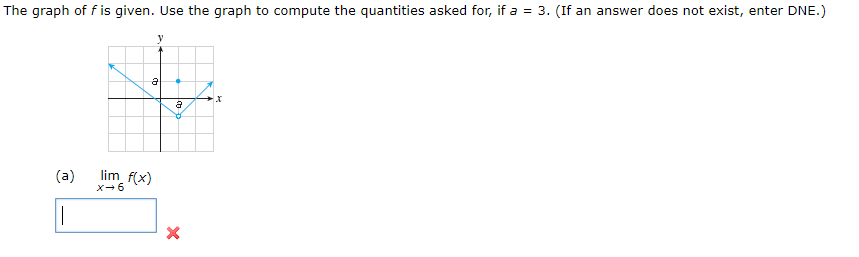# The graph of f is given. Use the graph to compute the quantities asked for, if a 3. (If an answer does not exist, enter DNE.)ylim f(x)(a)x6X

Questionhelp_outlineImage TranscriptioncloseThe graph of f is given. Use the graph to compute the quantities asked for, if a 3. (If an answer does not exist, enter DNE.) y lim f(x) (a) x6 X fullscreen
check_circleExpert Solution
Step 1

Given information:

The graph of the given function f is shown below:

Step 2

Calculation:

Since, the given function is continuous at x=6. so the...

### Want to see the full answer?

See Solution

#### Want to see this answer and more?

Solutions are written by subject experts who are available 24/7. Questions are typically answered within 1 hour*

See Solution
*Response times may vary by subject and question
Tagged in

### Calculus